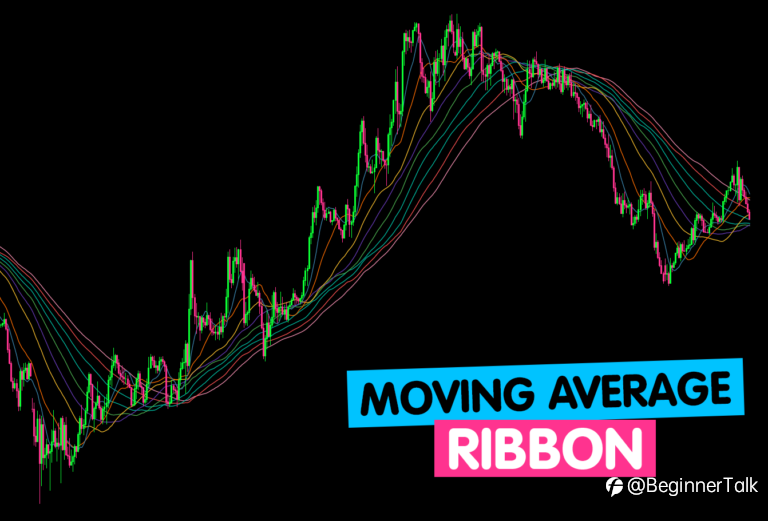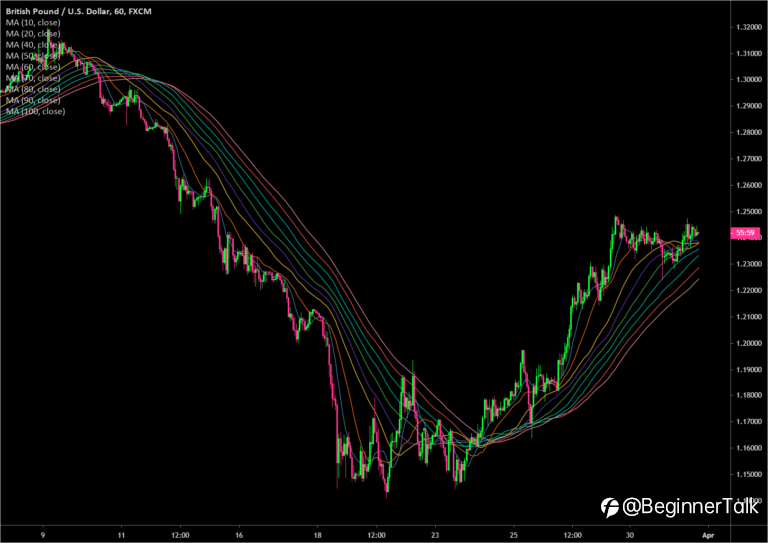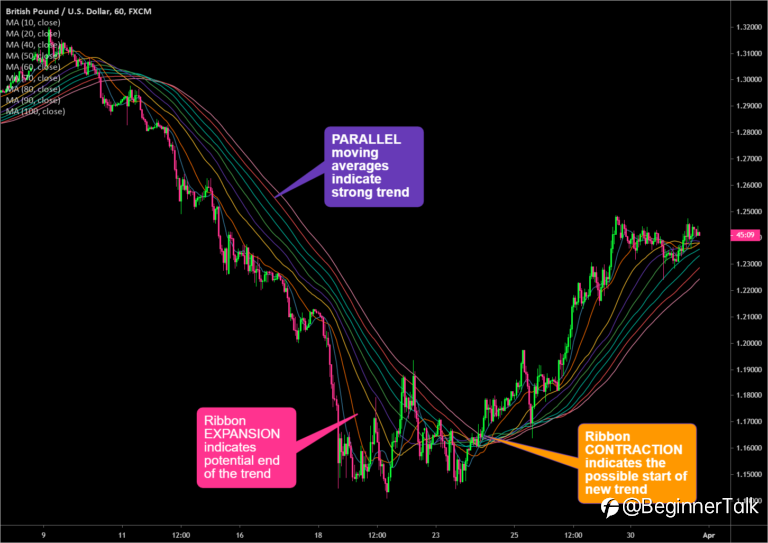Note

# How to Analyze Trends With Moving Average Ribbons

· View 232

What is a moving average ribbon?

A moving average ribbon is a series of moving averages of different lengths plotted on a chart.

The basic idea behind the concept of “moving average ribbons” is that instead of using one or two moving averages on a chart, you are using a bunch of moving averages, usually between 6 to 16 moving averages (or more).

All on the same chart.

Let’s take a look at an example…Traders can determine the strength of a trend by looking at the smoothness of the ribbon, as well as identify key areas of support or resistance by looking at the price in relation to the ribbon.

## How to Set Up a Moving Average Ribbon

A common question is “How many moving averages do I use?”

It really depends on the trader.

Some traders like to use six to eight simple moving averages (SMA) set at 10-period intervals, such as the 10, 20, 30, 40, 50, and 60-day SMAs.Other traders like to set up with SIXTEEN (or more) simple moving averages varying from a 50-day to a 200-day SMA and everything in between.

The argument for using longer-term MAs is that it gives a more accurate look at the overall trend.

Then other traders like to use exponential moving averages instead of simple moving averages.

So it’s really a matter of preference.

The responsiveness of the moving average ribbon can be adjusted by:

• Changing the number of time periods used in the moving average
• Changing the type of moving average from a simple moving average (SMA) to an exponential moving average (EMA)

The shorter the number of periods used when selecting which MAs to add to your chart, the more sensitive the moving average ribbon is to slight price changes.Using moving averages with larger numbers of periods (like 200) are less sensitive and smoother.

## How to Trade with Moving Average Ribbons

### 1. An EXPANDING moving average ribbon signals the potential end of a trend.

When the moving averages start widening out and separating, also known as ribbon “expansion”, this signals that that recent price direction has reached an extreme and could be the end of a trend.

Think of each moving average as a magnet and they’re attracted to each other.They do not want to be too far apart from each other for too long. So when they are, they will want to close that distance.

### 2. A CONTRACTING moving average ribbon signals a possible change in trend.

When the moving averages start to converge and get closer to each other, also known as ribbon “contraction”, a trend change has possibly started.

After an extreme move in price in one direction, you will notice shorter-term moving averages converge first. The longer-term moving averages will slowly converge.

### 3. A PARALLEL moving average ribbon signals a strong trend.

When the moving average ribbons are parallel and evenly spaced, this means that the current trend is strong.

All the moving averages are in “agreement” since they are moving together.

## Keep an Eye on the Spacing Between the Moving Averages

Some traders make the mistake of only paying attention when the moving averages “cross over” or “twist”.

While it is important to monitor when the short-term moving averages cross above (or below) the long-term moving averages, it’s also important to monitor the SPACING between the moving averages.

The positioning of short-term moving averages relative to long-term moving average shows the DIRECTION of the trend (down, neutral, up).

The spacing between the moving averages shows the STRENGTH of the trend (weak, neutral, strong).

## Moving Average Ribbon Example

Let’s take a look at a moving average ribbon applied to GBP/USD on a 1-hour chart.Can you see the trend changes?In the chart above, you can easily identify bullish or bearish trends by looking at when the moving averages start to cross over or “twist” lower or higher.

Ribbon expansion or the widening of spacing between the moving averages suggests the end of the current trend.

Ribbon contraction or the narrowing of spacing between the moving averages suggests the start of a new trend.